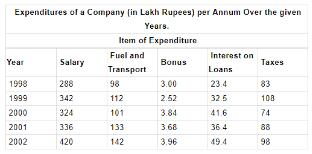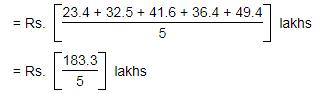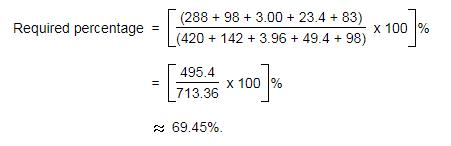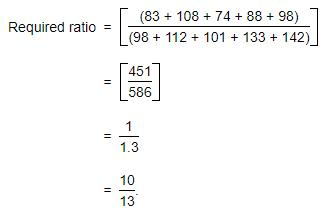Courses

# Test: Arun Sharma Based Level 2: Table Charts

## 5 Questions MCQ Test IBPS Clerk Prelims - Study Material, Mock Tests | Test: Arun Sharma Based Level 2: Table Charts

Description
This mock test of Test: Arun Sharma Based Level 2: Table Charts for UPSC helps you for every UPSC entrance exam. This contains 5 Multiple Choice Questions for UPSC Test: Arun Sharma Based Level 2: Table Charts (mcq) to study with solutions a complete question bank. The solved questions answers in this Test: Arun Sharma Based Level 2: Table Charts quiz give you a good mix of easy questions and tough questions. UPSC students definitely take this Test: Arun Sharma Based Level 2: Table Charts exercise for a better result in the exam. You can find other Test: Arun Sharma Based Level 2: Table Charts extra questions, long questions & short questions for UPSC on EduRev as well by searching above.
QUESTION: 1

### Study the following table and answer the questions based on it.What is the average amount of interest per year which the company had to pay during this period?

Solution:

Average amount of interest paid by the Company during the given period= Rs. 36.66 lakhs.

QUESTION: 2

### Study the following table and answer the questions based on it.The total amount of bonus paid by the company during the given period is approximately what percent of the total amount of salary paid during this period?

Solution:

[(300 + 2.52 + 3.84 + 3.68 + 3.96)*100]/[288 + 342 + 324 + 336 + 420]
= (17/1710)*100
which is approximately equal to 1.

QUESTION: 3

### Study the following table and answer the questions based on it.Total expenditure on all these items in 1998 was approximately what percent of the total expenditure in 2002?

Solution:QUESTION: 4

Study the following table and answer the questions based on it.The total expenditure of the company over these items during the year 2000 is?

Solution:

Total expenditure of the Company during 2000
= Rs. (324 + 101 + 3.84 + 41.6 + 74)lakhs
= Rs. 544.44 lakhs.

QUESTION: 5

Study the following table and answer the questions based on it.The ratio between the total expenditure on Taxes for all the years and the total expenditure on Fuel and Transport for all the years respectively is approximately?

Solution: